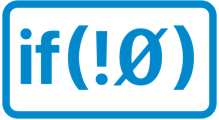# Replicate the Xojo "Assigns" feature in a Swift Program

As most of you know, I am working on a BASIC -> Swift translator, and one of the handy features that Xojo (for example) has, the Swift does no is the ASSIGNS feature.
Here is a snippet to demonstrate how to get that feature

BASIC

``````Sub myRoutine(var1 as String, var2 as Double, assigns Xyz as integer)
// do stuff
End sub
``````

SWIFT

``````private enum myRoutine {
subscript(_ var1 : String , _ var2 : Double) -> Int? {
get { return nil }
set { let Xyz = newValue!
let x : Int = 3 // THIS VARIABLE IS NEVER CHANGED [PATCH]
print(x)
}
}
}
``````

An ENUM… go figurethanks to @ JumhynFrederick Kellison-Linn on the Swift Forum for this idea.

1 Like

Turns out the ENUM version doesn’t work… it is Immutable… so not much goodThis one however DOES work

``````private Sub myRoutine(var1 as String, var2 as String, assigns Xyz as integer)
var x as integer =3
print(x)
End sub
sub test()
myRoutine("A","B")=3
end sub
``````

becomes

``````private var myRoutine = _myRoutine()
struct _myRoutine {
subscript(_ var1 : String , _ var2 : String) -> Int? {
get { return nil }
set {
let Xyz = newValue!
let x : Int = 3
print(x)
}
}
}
func test() {
myRoutine["A" , "B"] = 3
}
``````